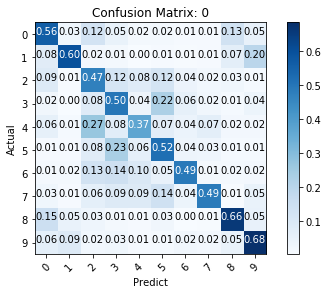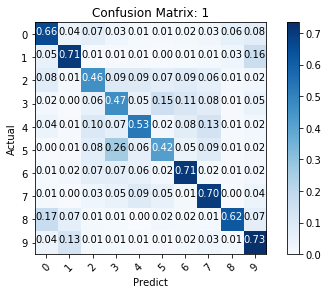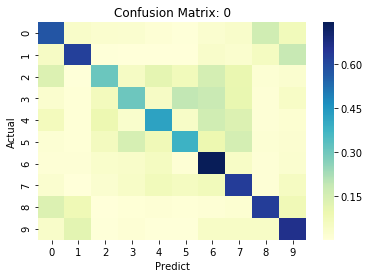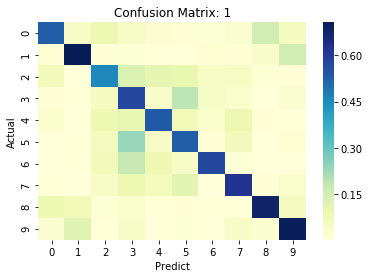# PyCM with Torchbearer¶

In this example, we show how to use the built in PyCM callback to create confusion matrices with torchbearer using the PyCM library.

Note: The easiest way to use this tutorial is as a colab notebook, which allows you to dive in with no setup. We recommend you enable a free GPU with

Runtime   →   Change runtime type   →   Hardware Accelerator: GPU

## Install Torchbearer¶

First we install torchbearer if needed.

In :
try:
import torchbearer
except:
!pip install -q torchbearer
import torchbearer

print(torchbearer.__version__)

0.4.0.dev


## Install PyCM¶

Next we install PyCM if needed.

In :
try:
import pycm
except:
!pip install -q pycm
import pycm


## A Simple Model¶

First we set up the simple CIFAR-10 model from the quickstart and load the data.

In :
import torch
import torchvision
from torchvision import transforms

from torchbearer.cv_utils import DatasetValidationSplitter

BATCH_SIZE = 128

normalize = transforms.Normalize(mean=[0.485, 0.456, 0.406],
std=[0.229, 0.224, 0.225])

transform=transforms.Compose([transforms.ToTensor(), normalize]))
splitter = DatasetValidationSplitter(len(dataset), 0.1)
trainset = splitter.get_train_dataset(dataset)
valset = splitter.get_val_dataset(dataset)

traingen = torch.utils.data.DataLoader(trainset, pin_memory=True, batch_size=BATCH_SIZE, shuffle=True, num_workers=0)
valgen = torch.utils.data.DataLoader(valset, pin_memory=True, batch_size=BATCH_SIZE, shuffle=True, num_workers=0)

transform=transforms.Compose([transforms.ToTensor(), normalize]))
testgen = torch.utils.data.DataLoader(testset, pin_memory=True, batch_size=BATCH_SIZE, shuffle=False, num_workers=0)

import torch.nn as nn

class SimpleModel(nn.Module):
def __init__(self):
super(SimpleModel, self).__init__()
self.convs = nn.Sequential(
nn.Conv2d(3, 16, stride=2, kernel_size=3),
nn.BatchNorm2d(16),
nn.ReLU(),
nn.Conv2d(16, 32, stride=2, kernel_size=3),
nn.BatchNorm2d(32),
nn.ReLU(),
nn.Conv2d(32, 64, stride=2, kernel_size=3),
nn.BatchNorm2d(64),
nn.ReLU()
)

self.classifier = nn.Linear(576, 10)

def forward(self, x):
x = self.convs(x)
x = x.view(-1, 576)
return self.classifier(x)

Files already downloaded and verified


## PyCM to_pyplot¶

Let's first have a look at how we can generate and plot a confusion matrix for our validation data, during the running of a Trial.

In :
%matplotlib inline
import torch
device = 'cuda' if torch.cuda.is_available() else 'cpu'

import torch.optim as optim

import torchbearer
from torchbearer import Trial
from torchbearer.callbacks import PyCM

cm = PyCM().on_val().to_pyplot(normalize=True, title='Confusion Matrix: {epoch}')

model = SimpleModel()
loss = nn.CrossEntropyLoss()

trial = Trial(model, optimizer, loss, metrics=['acc', 'loss'], callbacks=[cm]).to(device)
trial.with_generators(train_generator=traingen, val_generator=valgen)
history = trial.run(epochs=2, verbose=1)In the above code, we use string formatting to create a unique title for each confusion matrix and normalize the matrix. The available keys for formatting are anything in state, for the full list of keys, see the docs. The code used for the to_pyplot method is taken from the PyCM documentation example 2.

## to_file¶

Suppose we want to save our PyCM object to a file for analysis later. We can do this with the various to_XXX_file type methods in the PyCM object. Each method is based on one of the available save_XXX methods from PyCM, which can be found here. In this guide we'll have a look at two of the available methods, to_obj_file and to_html_file.

### obj¶

To save our matrices to obj files we again create a callback, this time just calling the to_obj_file method. We can again use string formatting here to save an individual file for each epoch.

In :
import torch
device = 'cuda' if torch.cuda.is_available() else 'cpu'

cm = PyCM().on_val().to_obj_file('cm.{epoch}')

model = SimpleModel()
loss = nn.CrossEntropyLoss()

trial = Trial(model, optimizer, loss, metrics=['acc', 'loss'], callbacks=[cm]).to(device)
trial.with_generators(train_generator=traingen, val_generator=valgen)
history = trial.run(epochs=5, verbose=1)


We can then simply use the PyCM library to load in our confusion matrices.

In :
from pycm import ConfusionMatrix, Compare

epoch_0 = ConfusionMatrix(file=open('cm.0.obj', 'r'))
epoch_1 = ConfusionMatrix(file=open('cm.1.obj', 'r'))
epoch_2 = ConfusionMatrix(file=open('cm.2.obj', 'r'))
epoch_3 = ConfusionMatrix(file=open('cm.3.obj', 'r'))
epoch_4 = ConfusionMatrix(file=open('cm.4.obj', 'r'))

print(Compare({"0": epoch_0, "1": epoch_1, "2": epoch_2, "3": epoch_3, "4": epoch_4}))

Best : 4

Rank     Name Class-Score     Overall-Score
1        4    30.95           4.48333
2        3    30.1            4.11667
3        2    29.8            3.86667
4        1    27.65           3.7
5        0    26.5            3.5



### HTML¶

To save to an HTML file we use the same approach as above.

In :
import torch
device = 'cuda' if torch.cuda.is_available() else 'cpu'

cm = PyCM().on_val().to_html_file('cm.{epoch}')

model = SimpleModel()
loss = nn.CrossEntropyLoss()

trial = Trial(model, optimizer, loss, metrics=['acc', 'loss'], callbacks=[cm]).to(device)
trial.with_generators(train_generator=traingen, val_generator=valgen)
history = trial.run(epochs=5, verbose=1)


This time, to view our confusion matrix we need to open a web browser.

Here's a link to open the confusion matrix from the last epoch

Note: This may not work in Colab but you can always just download the file and open it on your own machine.

## Custom Handlers¶

Suppose we wish to follow some of the examples from the PyCM docs. We can do this with torchbearer by creating a custom handler. Let's have a look at how we can plot with seaborn and pandas using example 7.

We first install some dependencies.

In :
try:
import seaborn as sns
except:
!pip install -q seaborn
import seaborn as sns

try:
from pandas import DataFrame
except:
!pip install -q pandas
from pandas import DataFrame


Next, we create our handler (a function of cm and state that does something with the confusion matrix) and a callback that will run it using the with_handler method.

In :
%matplotlib inline
import matplotlib.pyplot as plt

def to_pandas_seaborn(normalize=False,title='Confusion matrix',annot=False,cmap="YlGnBu"):
def handler(cm, state):
plt.figure()
string_state = {str(key): state[key] for key in state.keys()}  # For string formatting
if normalize == True:
df = DataFrame(cm.normalized_matrix).T.fillna(0)
else:
df = DataFrame(cm.matrix).T.fillna(0)
ax = sns.heatmap(df,annot=annot,cmap=cmap)
ax.set_title(title.format(**string_state))
ax.set(xlabel='Predict', ylabel='Actual')
plt.show()
return handler

from torchbearer.callbacks import PyCM
cm = PyCM().on_val().with_handler(to_pandas_seaborn(normalize=True, title='Confusion Matrix: {epoch}'))


Now all that remains is to run a trial with our callback to see the output.

In :
import torch
device = 'cuda' if torch.cuda.is_available() else 'cpu'

import torch.optim as optim

import torchbearer
from torchbearer import Trial

model = SimpleModel()